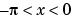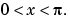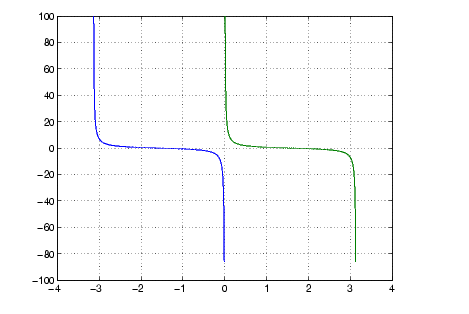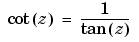MATLAB Function Referencecot

Cotangent of an argument in radians

Syntax

• ```Y = cot(X)
```

Description

The `cot` function operates element-wise on arrays. The function's domains and ranges include complex values. All angles are in radians.

```Y = cot(X) ``` returns the cotangent for each element of `X.`

Examples

Graph the cotangent the domainsand• ```x1 = -pi+0.01:0.01:-0.01;
x2 = 0.01:0.01:pi-0.01;
plot(x1,cot(x1),x2,cot(x2)), grid on```

Definition

The cotangent can be defined as

•Algorithm

`cot` uses FDLIBM, which was developed at SunSoft, a Sun Microsystems, Inc. business, by Kwok C. Ng, and others. For information about FDLIBM, see http://www.netlib.org.

See Also

`acot`, `acoth`, `cotd`, `coth`

© 1994-2005 The MathWorks, Inc.